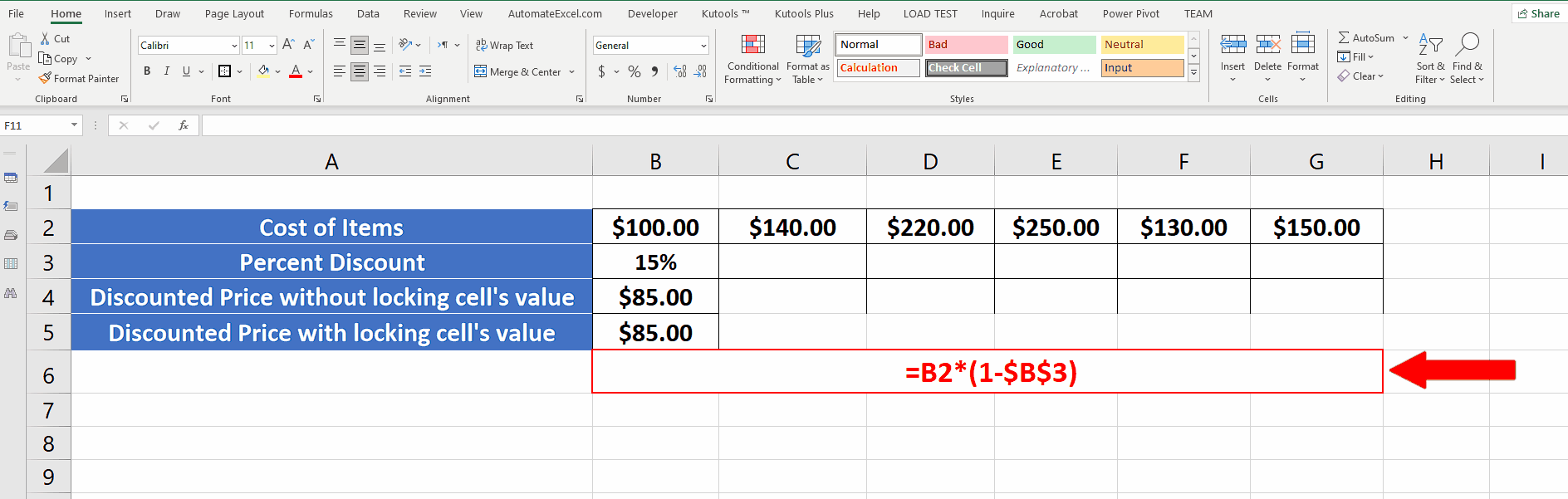# How to lock a cell in Excel formula

In this tutorial we’ll learn how to lock the cell or create an absolute reference to that cell in Excel formula by following this simple step.Excel provides a vast variety of formulas, functions and tools to perform simple to complex mathematical calculations. Quite often we need to use the value from the same cell in multiple calculations and that’s when we need to lock that cell’s value or make an absolute reference to that cell in a formula so that it doesn’t change while dragging down the formula.

### Step 1 – Use F4 shortcut key to lock the cell’s value in formula– The simplest of all methods is to write the formula and use the F4 shortcut key while keeping the cursor on the cell’s reference. This will add \$ sign before and after the cell reference which means that an absolute reference to that cell’s value will be used in this formula. In other words, the cell’s value is being locked now and it won’t change while dragging down the formula along the row or column as shown above.
– If we don’t use the absolute reference incorrect results will be obtained.